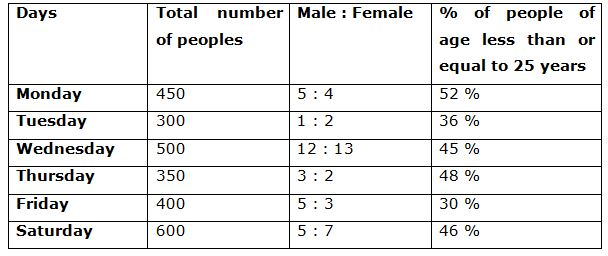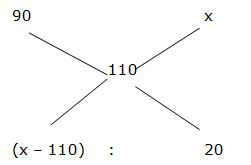# IBPS RRB Clerk Mains Quantitative Aptitude Questions 2019 (Day-09)

Dear Aspirants, Our IBPS Guide team is providing new series of Reasoning Questions for IBPS RRB Clerk Mains 2019 so the aspirants can practice it on a daily basis. These questions are framed by our skilled experts after understanding your needs thoroughly. Aspirants can practice these new series questions daily to familiarize with the exact exam pattern and make your preparation effective.

Check here for IBPS RRB PO Mains Mock Test 2019

Check here for IBPS RRB Clerk Mains Mock Test 2019

Check here for IBPS PO Prelims Mock Test 2019

### Click Here to Subscribe Crack High Level Puzzles & Seating Arrangement Questions PDF 2019 Plan

[WpProQuiz 7209]

1) 8 years ago, the age of the father and son is in the ratio of 5: 2. 7 years hence, the age of the father and his son is in the ratio of 13: 7. The average Present age of the father, mother, son and daughter is 38. The difference between the age of the mother and her daughter is 34. Then find the present age of the daughter?

a) 20 years

b) 18 years

c) 14 years

d) 16 years

e) None of these

2) If Ragu purchased some goods for Rs. 2700, and sold one-third of these goods at a loss of 10% then at what gain % should the remaining goods be sold to gain 10% on the whole transaction?

a) 24 %

b) 20 %

c) 15 %

d) 18 %

e) None of these

3) The speed of a boat in still water is 24 km/hr and the speed of the stream is 8 km/hr. It takes a total 20 hours to row upstream from point X to Point Y and downstream from Point Y to Point Z. If the distance from X to Y is one third of the distance between Y and Z. What is the total distance travelled by the boat (both upstream and downstream)?

a) 456 km

b) 424 km

c) 512 km

d) 578 km

e) None of these

4) A student gets 40 % marks and fails by 30 marks. Another student gets 52% marks so he gets 78 marks more than the passing marks. Find the passing marks in the examination?

a) 320

b) 430

c) 360

d) 390

e) None of these

5) A can complete a piece of work in 8 days while B can complete the same work in 12 days. They work together for 3 days. Then A quits the work. In how many days will B now be able to finish the remaining work?

a) 3 days

b) 5 days

c) 4 ½ days

d) 2 ¾ days

e) None of these

Directions (6 – 10): Study the following information carefully and answer the given questions:

Following table shows the total number of peoples went for a science conference in different days in a week and the ratio of male and female among them and the percentage of people of age less than or equal to 25 years.6) Total number of male went for a science conference in Monday and Wednesday together is what percentage of total number of female went for a science conference in Tuesday and Friday together?

a) 120 %

b) 130 %

c) 140 %

d) 100 %

e) 110 %

7) Find the difference between the total number of people whose age is above 25 years went for a conference in Thursday to that of Saturday?

a) 142

b) 128

c) 116

d) 164

e) None of these

8) Out of the total number of people whose age is less than or equal to 25 years went for a science conference in Wednesday, 44 % are females, then find the total number of females whose age is above 25 years went for a science conference in Wednesday?

a) 152

b) 144

c) 135

d) 161

e) None of these

9) Find the average number of males went for a science conference in Monday, Tuesday, Wednesday and Thursday together?

a) 350

b) 200

c) 400

d) 250

e) 300

10) Total number of peoples went for a science conference in Tuesday and Saturday together is what percentage more than the total number of peoples went for a science conference in Friday?

a) 125 %

b) 150 %

c) 75 %

d) 100 %

e) 200 %

Directions ( 1-5) :

8 years ago, the ratio of age of the father and his son = 5: 2 (5x, 2x)

7 years hence, the ratio of age of the father and his son = 13: 7

According to the question,

(5x + 15)/(2x + 15) = (13/7)

35x + 105 = 26x + 195

9x = 90

X = 10

The present age of the father and his son = (5x + 8), (2x + 8) = 58, 28

The average Present age of the father, mother, son and daughter = 38

Total Present age of the father, mother, son and daughter = 38*4 = 152

Total present age of the mother and her daughter = 152 – 86 = 66

Let the present age of mother and daughter be A and B,

A + B = 66 —>(1)

A – B = 34 —>(2)

By solving the equation (1) and (2),

A = 50, B = 16

The present age of daughter = 16 years

Shortcut:

One-third of these goods at a loss of 10% and remaining will be two third goods.

So, the ratio = (1/3): (2/3) = 1 : 2= > (x – 110)/20 = ½

= > x – 110 = 10

= > x = 120 %

So, 2/3rd of the goods will be sold at 20 % profit

The speed of a boat in still water = 24 km/hr

The speed of the stream = 8 km/hr

Speed of upstream = 24 – 8 = 16 km/hr

Speed of downstream = 24 + 8 = 32 km/hr

The distance from X to Y is one third of the distance between Y and Z

= > Distance(X to Y) = 1/3 * Distance(Y to Z)

Distance (Y to Z) is,

X/ (3*16) + X/32 = 20

X/48 +X/32 =20

X = 12*32 = 384 km

Distance from X to Y = 384*(1/3) = 128 km

Total Distance = 384 + 128

=> 512 km

Let total marks be x,

(40/100)*x + 30 = (52/100)*x – 78

(12/100)*x = 108

X= 108*(100/12) = 900

Passing mark = (40/100)*900 + 30 = 390

A’s one day work = 1/8

B’s one day work = 1/12

(A + B)’s one day work = (1/8) + (1/12) = 5/24

(A + B)’s 3 day work = (5/24)*3 = 5/8

Remaining work = 1 – (5/8) = 3/8

B can finish the remaining work in = (3/8)*12 = 9/2 = 4 ½ days

Directions (6-10) :

Total number of male went for a science conference in Monday and Wednesday together

= > 450*(5/9) + 500*(12/25)

= > 250 + 240 = 490

Total number of female went for a science conference in Tuesday and Friday together

= > 300*(2/3) + 400*(3/8)

= > 200 + 150 = 350

Required % = (490/350)*100 = 140 %

The total number of people whose age is above 25 years went for a conference in Thursday

= > 350*(52/100) = 182

The total number of people whose age is above 25 years went for a conference in Saturday

= > 600*(54/100) = 324

Required difference = 324 – 182 = 142

The total number of females went for a science conference in Wednesday

= > 500*(13/25) = 260

The total number of females whose age is less than or equal to 25 years went for a science conference in Wednesday

= > 500*(45/100)*(44/100) = 99

The total number of females whose age is above 25 years went for a science conference in Wednesday

= > 260 – 99 = 161

The total number of males went for a science conference in Monday, Tuesday, Wednesday and Thursday together

= > 450*(5/9) + 300*(1/3) + 500*(12/25) + 350*(3/5)

= > 250 + 100 + 240 + 210

= > 800

Required average = 800/4 = 200

Total number of peoples went for a science conference in Tuesday and Saturday together

= > 300 + 600 = 900

Total number of peoples went for a science conference in Friday

= > 400

Required % = [(900 – 400)/400]*100 = 125 %